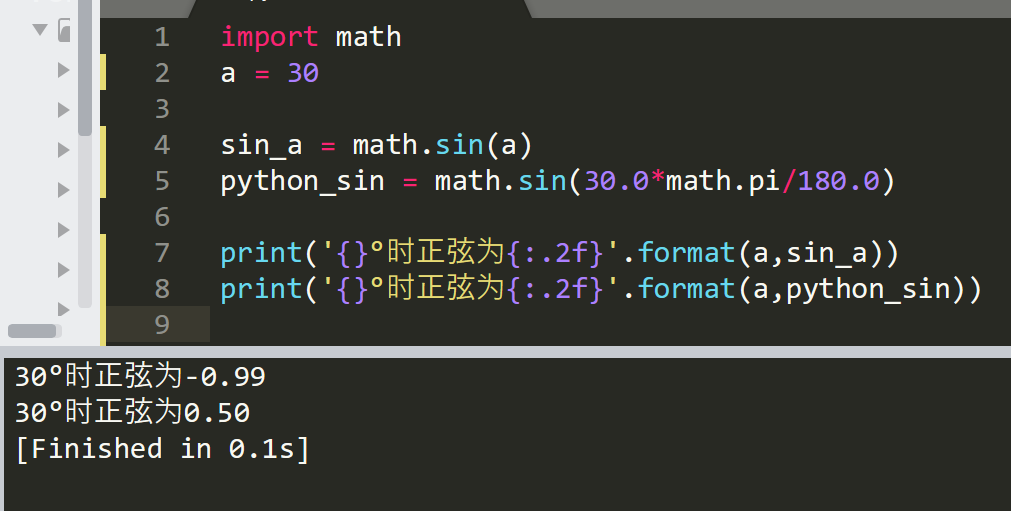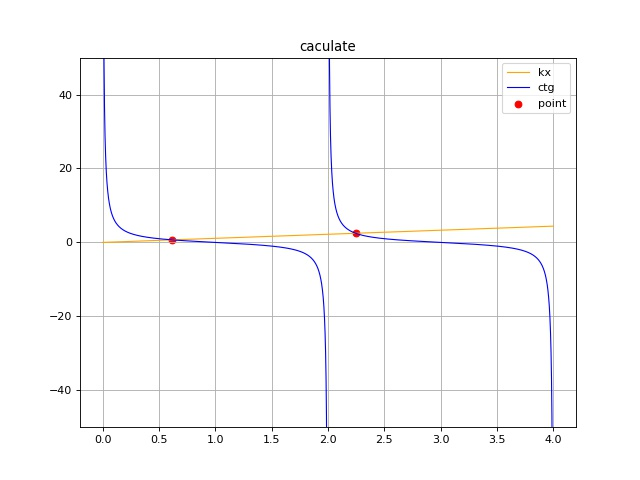• 主要介绍了使用Python三角函数公式计算三角形夹角案例，具有很好参考价值，希望对大家有所帮助。一起跟随小编过来看看吧
• 最近练习碰到一个问题，使用python计算三角函数时，结果与计算器计算结果一样，查询了资料发现差异问题为： python math中三角函数数值是弧度，而计算器中数值是角度 通过以下方法可以做确认： 计算器计算...
最近练习碰到一个问题，使用python计算三角函数时，结果与计算器计算结果一样，查询了资料发现差异的问题为：
python math中三角函数中的数值是弧度，而计算器中的数值是角度
通过以下方法可以做确认：展开全文• 主要介绍了Python计算三角函数之asin()方法的使用,是Python入门的基础知识,需要的朋友可以参考下
• 主要介绍了用Python计算三角函数之atan()方法的使用,是Python入门的基础知识,需要的朋友可以参考下
• 主要介绍了用Python计算三角函数之acos()方法的使用,是Python学习中的基础知识,需要的朋友可以参考下
• python是用来导入模块，在python模块库中有着大量模块可供使用，要想使用这些文件需要需要用import语句将指定模块导入到当前程序中。所以使用函数，需要使用math模块。 [gyz@archlinux job]...
 有错的地方，或者不同意见的，烦请留言，或者发邮箱。

邮箱地址：1746902011@qq.com

—————————————————————————————————

python是用来导入模块的，在python模块库中有着大量的模块可供使用，要想使用这些文件需要需要用import语句将指定模块导入到当前程序中。所以使用函数，需要使用math模块。

[gyz@archlinux job]\$ python
Python 3.7.1 (default, Oct 22 2018, 10:41:28)
[GCC 8.2.1 20180831] on linux
Type "help", "copyright", "credits" or "license" for more information.
>>> import math


1，自然指数函数，即e^x

>>> math.exp(0)
1.0
>>> math.exp(1)
2.718281828459045

2，对数函数，即log(x)

在python中是log(x,a)，即以a为底数，

当a=10时，

>>> math.log(1,10)
0.0
>>> math.log(10,10)
1.0
>>> math.log(100,10)
2.0

当a=e时，（在python中e=math.e）

>>> math.log(1,math.e)
0.0
>>> math.log(math.e,math.e)
1.0

3，正弦函数，即sin(x)

在python中，圆周率用math.pi表示

>>> math.sin(0)
0.0
>>> math.sin(math.pi/2)
1.0

4，余弦函数，即cos(x)

>>> math.cos(0)
1.0
>>> math.cos(math.pi)
-1.0

5，绝对值，即|x|

>>> abs(10)
10
>>> abs(-10)
10
>>> 

6，求平方根

>>> math.sqrt(4)
2.0
>>> math.sqrt(9)
3.0


7，向上取整函数

>>> math.ceil(4.1)
5
>>> math.ceil(-4.1)
-4

8，向下取整函数

>>> math.floor(4.1)
4
>>> math.floor(-4.1)
-5


9，取一组数中最小值的函数

>>> min(1,2,3)
1
>>> min(2,3,4)
2
>>> min(3,4,5)
3

10，取一组数中最大值的函数

>>> max(1,2,3)
3
>>> max(2,3,4)
4
>>> max(3,4,5)
5

参考1：http://www.runoob.com/python/python-numbers.html

参考2：http://www.iplaypy.com/jinjie/import.html


展开全文• 主要介绍了Python中计算三角函数之cos()方法的使用简介,是Python入门的基础知识,需要的朋友可以参考下
• # 函数的使用 # 什么是函数？封装了一段代码的功能模块，可以被重复使用多次 # 例如：数学中三角函数.... # def 函数名(参数列表): # 函数名称的命名规范： # 每个单词都是小写的，单词之间用_下划线链接 def eat()...
# -*- coding:utf-8 -*-

# 函数的使用
# 什么是函数？封装了一段代码的功能模块，可以被重复使用多次
# 例如：数学中三角函数....

# def 函数名(参数列表):
# 函数名称的命名规范：
# 每个单词都是小写的，单词之间用_下划线链接
def eat():
print('1.买菜')
print('2.洗菜')
print('3.炒菜')
print('4.吃饭')
print('5.刷完')

# 函数被调用的时候，才会执行
eat()

# 带有参数的函数
# 买水  需要给钱
# money 参数名要和参数的用处有关联，见名知意
# 必备参数 调用时，必须要传递的参数
def buy_water(money, name):

print(money,name)
# 调用的时候必须将两个参数进行传递
buy_water(10, '康师傅矿泉水')

# 缺省参数
# 这种参数可以不用传入，如果不传入，就是默认值，如果传入，time就是传入的值
def sleep(name,time=8):

print('{}睡了{}小时'.format(name,time))

sleep('小明',12)

# 传入的参数个数不确定
# 不定长参数，实际上传递进来之后，是一个元组，元组中存放的是数据
def print_number(*args):
print(type(args))
print(args)

print_number(1,2,3,4,5,6,7,8,9)

# 传入多个关键字参数
# 不定长的关键字参数，实际上传入是一个字典
def test(**kwargs):
print(type(kwargs))
print(kwargs)
# 调用时，必须按照key=value的形式传递参数
test(name='张三',age='22')

# 如果一个函数中有必备参数，缺省参数，不定长参数，关键字不定长参数，需要注意这几个参数的先后顺序
def total(name, age=10, *args, **kwargs):
print(name,age,args,kwargs)

total('小明', 22, 1,2,3,4,5,phone=110,sex='男')

# 函数中return有两个作用
# 1.返回函数执行的结果
def test1(a,b):

print('......')
# 如果一个函数没有return返回一个值，默认返回的就是None

return a + b

rs = test1(100,200)
print(rs)
# 2.结束函数的执行
def test2(a):
print('-------------')
if a == 10:
return
# return下方的代码就不会再执行了
print('**************')

test2(10)

# 3.在函数中，返回多个值,返回的多个值，是放在一个元组中的
def test3():

return 1,2,3,4,5,6,7,8,9

rs  = test3()

print(rs)


展开全文• 由于一些物理计算需要，我要用电脑将一个一次函数和一个三角函数（cotan）图像和交点画出来。 本例程使用库： numpy 强大科学计算库 matlibplot python绘图库 random 随机数产生库 由于计算机只能...
由于一些物理计算的需要，我要用电脑将一个一次函数和一个三角函数（cotan）的图像和交点画出来。

本例程使用到的库：

numpy   强大的科学计算库

matlibplot   python绘图库

random   随机数产生库

由于计算机只能计算离散的点，所以我们不太可能直接求得精确地交点值，所以只能求一个大致的值，我选择两个函数值相差值小于等于0.01的时候就认为是交点。

但是即使是这样，还是会在一个小范围内得到不止一个点，所以我们还需要进行数据清洗工作

由于我们只是表示出了cotan的两个区间，所以要在每个区间取一个点。

import numpy as np
import matplotlib.pyplot as plt
from random import randint

def get_ctg(i):
i = np.pi/2 * i
return np.cos(i)/np.sin(i)

x = np.linspace(0, 4, 100000)    #0到10 的 10000个点

x_l = []  #记录最终的xy值
y_l = []
n = 1

y1 = []
x1 = []
y2 = []
x2 = []
def get_y1():
for x_i in x:
y1.append(n * x_i)
x1.append(x_i)

def get_y2():
for x_i in x:
if(np.sin(x_i) != 0):
y = get_ctg(x_i)
y2.append(y)
x2.append(x_i)

#获得两个函数的大致交点
def get_point():
for x_i in x2:  #直接过滤掉等于0的点
y = n*x_i - get_ctg(x_i)
if(abs(y) <= 0.01):
x_l.append(x_i)
y_l.append(n*x_i)
#print("x_l=",x_l)
#print("y_l=",y_l)

#对交点数据进行清洗
def clean():
index = 0
if(len(x_l) > 2):
while(x_l[index] < 2):
index = index+1
num = index
#然后从0 - index中随机取一个点
index = randint(0, index-1)
x_l = x_l[index]
y_l = y_l[index]
x_l = x_l[num]
y_l = y_l[num]
#删除其他元素
""""
for i in range(1, len(x_l)):
del y_l[i]
del x_l[i]
"""

def get_image(y1, y2):
#绘图
fig = plt.figure(figsize=[8, 6], dpi=80)
plt.grid()
plt.plot(x1, y1, color="orange", linewidth=1.0, linestyle="-", label="kx")
plt.plot(x2[:len(x2)//2], y2[:len(x2)//2], color="blue", linewidth=1.0, linestyle="-", label="ctg")
plt.plot(x2[len(x2)//2:], y2[len(x2)//2:], color="blue", linewidth=1.0, linestyle="-")
plt.scatter(x_l[:2], y_l[:2], color="red", label="point")
plt.title("caculate")
plt.ylim(-50, 50)
#将坐标原点置中

plt.legend()
plt.savefig("caculate.jpg", dpi=80)
plt.show()

if __name__ == "__main__":
#n = float(input("请输入nig的值："))
n = float(input("请输入一次函数的斜率："))
get_y1()
get_y2()
get_point()
clean()
print("第一个点的x=", x_l)
print("第一个点的y=", y_l)
print("第二个点的x=", x_l)
print("第二个点的y=", y_l)
get_image(y1, y2)

最后画出的图像如下图所示展开全文• 使用Python玩转高等数学：三角函数三角函数开始，我们使用matplotlib绘制函数图像，matplotlib是图形绘制库，使用matplotlib可以方便绘制函数图形，以及直方图、条形图、散点图等统计图形。 matplotlib绘图...
• 最近其实是在看《数学女孩》书籍，看着看着觉得中间故事情节确实是累赘了。切换一下思维，找来了之前看过...简单使用Python实现如下：  1 #!/usr/bin/python   2   3 pi = 3.1415926  4   5 def f
• 参考链接： Python数学math函数 3(三角函数和角函数) 数学函数 函数返回值 ( 描述 )abs(x)返回数字绝对值，如abs(-10) 返回 10ceil(x)返回数字上入整数，如math.ceil(4.1) 返回 5cmp(x, y)如果 x < y ...
• 参考链接： Pythonnumpy.arcsin The following are code examples for showing how to use . They are extracted from open source Python projects. You can vote up the examples you like or vote down the ...
• 果不其然，在里面找到了我需要正弦函数、对数函数、自然数e、以及圆周率π，值得注意是 pi=π，在python中导入math模块就可以使用啦！要记得哦，是先导入math模块！如果不先导入math模块有什么结果呢，可看下图 ...
• 参考链接： Pythonnumpy.right_shift 参考链接： Pythonnumpy.arcsin The following are code examples for showing how to use . They are extracted from open source Python projects. You can vote up...
• Python可构造球三角函数 尽管存在针对诸如大地测量学等领域一系列球面三角函数库，但是这些库使用浮点数或最好使用有理数。 另一方面，可构造数字具有历史和几何意义，可以使用标尺和指南针“构造”这些数字。...
• # 函数的使用 # 什么是函数？封装了一段代码的功能模块，可以被重复多次使用 # 例如：数学中的三角函数 # def 函数名(参数列表)： # 函数名称的命名规范： # 每个单词都是小写，单词之间用_下划线连接 def eat(): ...
• 描述 atan2() 返回给定 X 及 Y 坐标值反正切值。 语法 以下是 atan2() 方法语法: ...以下展示了使用 atan2() 方法实例： #!/usr/bin/python import math print atan2(-0.50,-0.50) : , math.atan2(-0.50,
• 描述 sin()返回x弧度正弦值。...以下展示了使用sin()方法实例： #!/usr/bin/python import math print "sin(3) : ", math.sin(3) print "sin(-3) : ", math.sin(-3) print "sin(0) : ", math.sin(0) print
• 描述 tan() 返回x弧度正弦值。 语法 以下是 tan() 方法语法:...以下展示了使用 tan() 方法实例： #!/usr/bin/python import math print tan(3) : , math.tan(3) print tan(-3) : , math.tan(-3) print tan(0)
• 本期为如何使用Python语言，编写判断三角形的函数的实现方法，本文只是一个简单的实现方法，可以在此基础上进行扩展，使其实现的更好，同时在一些公司笔试面试题当中也是可能碰见的，但是一般是在面试一些需要编程...
• # 函数的使用 # 什么是函数？封装了一段代码的功能模块，可以被重复多次使用 # 例如：数学中的三角函数 # def 函数名(参数列表)： # 函数名称的命名规范： # 每个单词都是小写，单词之间用_下划线连接 def eat(): ...
• Python的numpy中常用函数的详细介绍 参考>>bolg https://blog.csdn.net/yipala/article/details/100133485 Numpy数学函数abs，sqrt()，square()，exp()，log()，sign()， 四舍五入函数，modf()，isnan()，is...numpy
• 编写一个函数，输入三个数，作为三角形三个边长，计算三角形面积。海伦公式：p=(x+y+z)/2 S=sqrt(p*(p-x)(p-y)(p-z)) def halen(x,y,z): p = 0.5 * (x + y + z) S = (p * (p - x) * (p - y) * (p - z)) ** 0.5......

# python三角函数的使用python 订阅